Analysis of Black Friday Shopping Trends via Machine Learning - Stack Abuse

# Analysis of Black Friday Shopping Trends via Machine Learning### Introduction

Wikipedia defines Black Friday as an informal name for the Friday following Thanksgiving Day in the United States, which is celebrated on the fourth Thursday of November. [Black Friday is] regarded as the beginning of America's Christmas shopping season [...].

In this article, we will try to explore different trends from the Black Friday shopping dataset. We will extract useful information that will answer questions such as: what gender shops more on Black Friday? Do the occupations of the people have any impact on sales? Which age group is the highest spender?

In the end, we will create a simple machine learning algorithm that predicts the amount of money that a person is likely to spend on Black Friday depending on features such as gender, age, and occupation.

The dataset that we will use in this article includes 550,000 observations about Black Friday, which are made in a retail store. The file can be downloaded at the following Kaggle link: Black Friday Case Study.

### Data Analysis

The first step is to import the libraries that we will need in this section:

import pandas as pd
import numpy as np
import matplotlib as pyplot
%matplotlib inline
import seaborn as sns


Next, we need to import our data.

data = pd.read_csv('E:/Datasets/BlackFriday.csv')


Let's see some basic information about our data!

data.info()


Output:

<class 'pandas.core.frame.DataFrame'>
RangeIndex: 537577 entries, 0 to 537576
Data columns (total 12 columns):
User_ID                       537577 non-null int64
Product_ID                    537577 non-null object
Gender                        537577 non-null object
Age                           537577 non-null object
Occupation                    537577 non-null int64
City_Category                 537577 non-null object
Stay_In_Current_City_Years    537577 non-null object
Marital_Status                537577 non-null int64
Product_Category_1            537577 non-null int64
Product_Category_2            370591 non-null float64
Product_Category_3            164278 non-null float64
Purchase                      537577 non-null int64
dtypes: float64(2), int64(5), object(5)
memory usage: 49.2+ MB


Looking at the data, we can conclude that our set possesses 12 different parameters: 7 numerical (integer and float) and 5 object variables. Furthermore, the dataset contains two short type variables: Product_Category_2 and Product_Category_3. We will see later how to handle this problem.

Ok, now we have a general picture of the data, let's print information about first five customers (first five rows of our DataFrame):

data.head()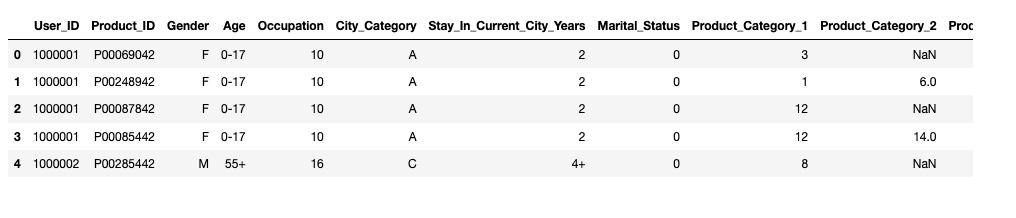The first question I want to ask from the beginning of this study, is it true that female customers are highly dominant in comparison to male customers? We will use the seaborn library and the countplot function to plot the number of male and female customers.

sns.countplot(data['Gender'])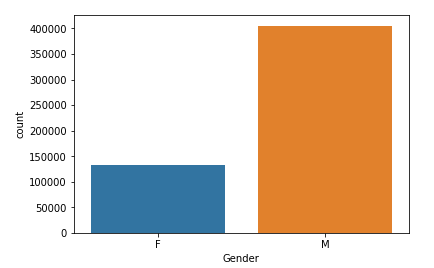Wow! The graph shows that there are almost 3 times more male customers than female customers! Why is that? Maybe male visitors are more likely to go out and buy something for their ladies when more deals are present.

Let's explore the Gender category a bit more. We want to see now distribution of gender variable, but taking into consideration the Age category. Once again countplot function will be used, but now with defined hue parameter.

sns.countplot(data['Age'], hue=data['Gender'])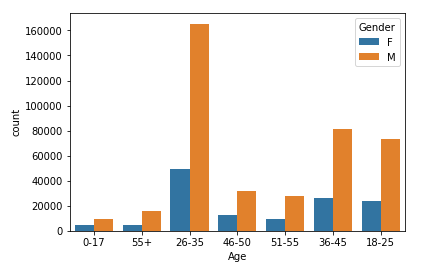From the figure above, we can easily conclude that the highest number of customers belong to the age group between 26 and 35, for both genders. Younger and older population are far less represented on Black Friday. Based on these results, the retail store should sell most of the products that target people in their late twenties to early thirties. To increase profits, the number of products targeting people around their thirties can be increased while the number of products that target the older or younger population can be reduced.

Next, we will use the describe function to analyze our categories, in terms of mean values, min and max values, standard deviations, etc...

data.describe()Further, below we analyze the User_ID column using the nunique method. From this we can conclude that in this specific retail store, during Black Friday, 5,891 different customers have bought something from the store. Also, from Product_ID category we can extract information that 3,623 different products are sold.

data['User_ID'].nunique()


Output:

5891

data['User_ID'].nunique()


Output:

3623


Now let's explore the Occupation category. The Occupation number is the ID number of occupation type of each customer. We can see that around 20 different occupations exist. But let's perform exact analysis. First, we need to create the function which will extract all unique elements from one column (to extract all different occupations).

We will use the unique function for that, from the numpy Python library.

def unique(column):
x = np.array(column)
print(np.unique(x))

print("The unique ID numbers of customers occupations:")
unique(data['Occupation'])


Output:

The unique ID numbers of costumers occupations:
[ 0  1  2  3  4  5  6  7  8  9 10 11 12 13 14 15 16 17 18 19 20]


As we can see, 21 different occupation ID's are registered during the shopping day.

The Occupation number could represent different professions of customers: for example, number 1 could be an engineer, number 2 - a doctor, number 3 an artist, etc.

It would be also interesting to see how much money each costumer group (grouped by occupation ID) spent. To do that, we can use a for loop and sum the spent money for each individual occupation ID:

occupations_id = list(range(0, 21))
spent_money = []
for oid in occupations_id:
spent_money.append(data[data['Occupation'] == oid]['Purchase'].sum())

spent_money


Output:

[625814811,
414552829,
233275393,
160428450,
657530393,
112525355,
185065697,
549282744,
14594599,
53619309,
114273954,
105437359,
300672105,
71135744,
255594745,
116540026,
234442330,
387240355,
60249706,
73115489,
292276985]


We have created the list spent_money, which includes summed quantities of dollars for the Occupations IDs - from 0 to 20. It may seem odd in the results that hundreds of millions of dollars are spent. But, keep in mind that our dataset includes 500,000 observations, so this is actually very likely. Or maybe the retail store is actually a big shopping mall. Another explanation for the huge sums of money spent by each occupation is that this data may represent the transactions for multiple Black Friday nights, and not just one.

Now, we have information about how much money is spent per occupation category. Let's now graphically plot this information.

import matplotlib.pyplot as plt; plt.rcdefaults()
import matplotlib.pyplot as plt

objects = ('0', '1', '2', '3', '4', '5','6','7','8','9','10', '11','12', '13', '14', '15', '16', '17', '18', '19', '20')
y_pos = np.arange(len(objects))

plt.bar(y_pos, spent_money, align='center', alpha=0.5)
plt.xticks(y_pos, objects)
plt.ylabel('Money spent')
plt.title('Occupation ID')

plt.show()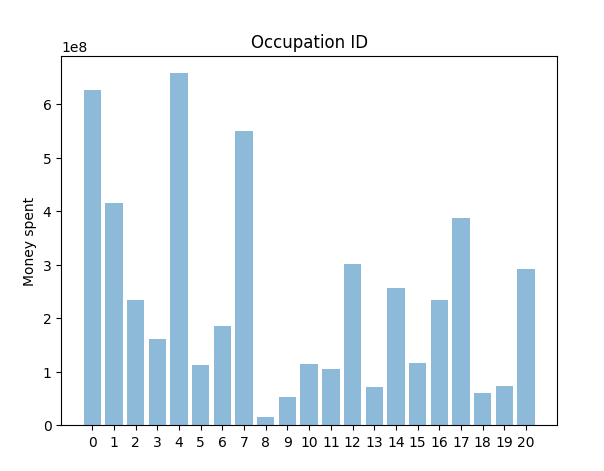It can be easily observed that people having occupations 0 and 4 spent the most money during Black Friday sales. On the other hand, the people belonging to the occupations with ID 18, 19, and especially occupation 8, have spent the least amount of money. It can imply that these groups are the poorest ones, or contrary, the richest people who don't like to shop in that kind of retail stores. We have a deficiency with information to answer that question, and because of that, we would stop here with the analysis of the Occupation category.

City_Category variable is the next one. This category gives us information about cities from which our customers are. First, let's see how many different cities do we have.

## Better understand your data with visualizations

•  30-day no-questions refunds
•  Beginner to Advanced
•  Updated regularly (update June 2021)
•  New bonus resources and guides
data['City_Category'].nunique()


Output:

3


Now, it will be interesting to see in percentages, what is the ratio of customers from each city. This information will be presented in the form of a colored pie chart. We can do so in 5 lines of code. Almighty Python, thank you! :)

explode = (0.1, 0, 0)
fig1, ax1 = plt.subplots(figsize=(11,6))
ax1.pie(data['City_Category'].value_counts(), explode=explode, labels=data['City_Category'].unique(), autopct='%1.1f%%')
plt.legend()
plt.show()It is evident from the pie chart that all the three cities are almost equally represented in the retail store during Black Fridays. Maybe the store is somewhere between these three cities, is easily accessible and has good road connections from these cities.

### Data Preprocessing for ML Algorithms

We have covered until now a few basic techniques for analyzing raw data. Before we can apply machine learning algorithms to our dataset, we need to convert it into a certain form that machine learning algorithms can operate on. The task of the learning algorithms will be to predict the value of the Purchase variable, given customer information as input.

The first thing that we need to do is deal with missing data in columns Product_Category_2 and Product_Category_3. We have only 30% of data inside Product_Category_3 and 69% of data inside Product_Category_2. 30% of real data is a small ratio, we could fill missing values inside this category with the mean of the existing values, but that means that 70% of data will be artificial, which could ruin our future machine learning model. The best alternative for this problem is to drop this column from further analysis. We will use drop function to do that:

data = data.drop(['Product_Category_3'], axis=1)


The column Product_Category_2 posses around 30% of missing data. Here it makes sense to fill missing values and use this column for fitting a machine learning model. We will solve this problem by inserting a mean value of the existing values in this column to the missing fields:

data['Product_Category_2'].fillna((data['Product_Category_2'].mean()), inplace=True)


Let's now check our data frame again:

data.info()


Output:

<class 'pandas.core.frame.DataFrame'>
RangeIndex: 537577 entries, 0 to 537576
Data columns (total 11 columns):
User_ID                       537577 non-null int64
Product_ID                    537577 non-null object
Gender                        537577 non-null object
Age                           537577 non-null object
Occupation                    537577 non-null int64
City_Category                 537577 non-null object
Stay_In_Current_City_Years    537577 non-null object
Marital_Status                537577 non-null int64
Product_Category_1            537577 non-null int64
Product_Category_2            537577 non-null float64
Purchase                      537577 non-null int64
dtypes: float64(1), int64(5), object(5)
memory usage: 45.1+ MB


The problem of missing values is solved. Next, we will remove the columns that do not help in the prediction.

User_ID is is the number assigned automatically to each customer, and it is not useful for prediction purposes.

The Product_ID column contains information about the product purchased. It is not a feature of the customer. Therefore, we will remove that too.

data = data.drop(['User_ID','Product_ID'], axis=1)
data.info()


Output:

<class 'pandas.core.frame.DataFrame'>
RangeIndex: 537577 entries, 0 to 537576
Data columns (total 9 columns):
Gender                        537577 non-null object
Age                           537577 non-null object
Occupation                    537577 non-null int64
City_Category                 537577 non-null object
Stay_In_Current_City_Years    537577 non-null object
Marital_Status                537577 non-null int64
Product_Category_1            537577 non-null int64
Product_Category_2            537577 non-null float64
Purchase                      537577 non-null int64
dtypes: float64(1), int64(4), object(4)
memory usage: 36.9+ MB


Our final selection is based on 9 columns - one variable we want to predict (the Purchase column) and 8 variables which we will use for training our machine learning model.

As we can see from the info table, we are dealing with 4 categorical columns. However, basic machine learning models are capable of processing numerical values. Therefore, we need to convert the categorical columns to numeric ones.

We can use a get_dummies Python function which converts categorical values to one-hot encoded vectors. How does it work? We have 3 cities in our dataset: A, B, and C. Let's say that a customer is from city B. The get_dummies function will return a one-hot encoded vector for that record which looks like this: [0 1 0]. For a costumer from city A: [1 0 0] and from C: [0 0 1]. In short, for each city a new column is created, which is filled with all zeros except for the rows where the customer belongs to that particular city. Such rows will contain 1.

The following script creates one-hot encoded vectors for Gender, Age, City, and Stay_In_Current_City_Years column.

df_Gender = pd.get_dummies(data['Gender'])
df_Age = pd.get_dummies(data['Age'])
df_City_Category = pd.get_dummies(data['City_Category'])
df_Stay_In_Current_City_Years = pd.get_dummies(data['Stay_In_Current_City_Years'])

data_final = pd.concat([data, df_Gender, df_Age, df_City_Category, df_Stay_In_Current_City_Years], axis=1)

data_final.head()


In the following screenshot, the newly created dummy columns are presented. As you can see, all categorical variables are transformed into numerical. So, if a customer is between 0 and 17 years old (for example), only that column value will be equal to 1, other, other age group columns will have a value of 0. Similarly, if it is a male customer, the column named 'M' will be equal to 1 and column 'F' will be 0.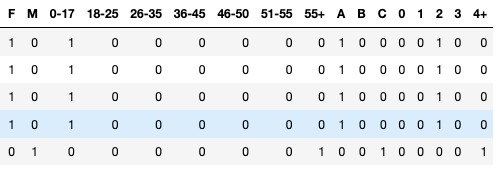Now we have the data which can be easily used to train a machine learning model.

### Predicting the Amount Spent

In this article, we will use one of the simplest machine learning models, i.e. the linear regression model, to predict the amount spent by the customer on Black Friday.

Linear regression represents a very simple method for supervised learning and it is an effective tool for predicting quantitative responses. You can find basic information about it right here: Linear Regression in Python

This model, like most of the supervised machine learning algorithms, makes a prediction based on the input features. The predicted output values are used for comparisons with desired outputs and an error is calculated. The error signal is propagated back through the model and model parameters are updating in a way to minimize the error. Finally, the model is considered to be fully trained if the error is small enough. This is a very basic explanation and we are going to analyze all these processes in details in future articles.

Enough with the theory, let's build a real ML system! First, we need to create input and output vectors for our model:

X = data_final[['Occupation', 'Marital_Status', 'Product_Category_2', 'F', 'M', '0-17', '18-25', '26-35', '36-45', '46-50', '51-55', '55+', 'A', 'B', 'C', '0', '1', '2', '3', '4+']]
y = data_final['Purchase']


Now, we will import the train_test_split function to divide all our data into two sets: training and testing set. The training set will be used to fit our model. Training data is always used for learning, adjusting parameters of a model and minimizing an error on the output. The rest of the data (the Test set) will be used to evaluate performances.

The script below splits our dataset into 60% training set and 40% test set:

from sklearn.model_selection import train_test_split

X_train, X_test, y_train, y_test = train_test_split(X, y, test_size=0.4)


Now it is time to import our Linear Regression model and train it on our training set:

from sklearn.linear_model import LinearRegression

lm = LinearRegression()
lm.fit(X_train, y_train)
print(lm.fit(X_train, y_train))


Output:

LinearRegression(copy_X=True, fit_intercept=True, n_jobs=None,
normalize=False)


Congrats people! Our model is trained. We can now print the intercept parameter value and values of all coefficients of our model, after the learning procedure:

print('Intercept parameter:', lm.intercept_)
coeff_df = pd.DataFrame(lm.coef_, X.columns, columns=['Coefficient'])
print(coeff_df)


Output:

Intercept parameter: 11224.23064289564
Coefficient
Occupation             8.110850
Marital_Status       -79.970182
Product_Category_2  -215.239359
F                   -309.477333
M                    309.477333
0-17                -439.382101
18-25               -126.919625
26-35                 67.617548
36-45                104.096403
46-50                 14.953497
51-55                342.248438
55+                   37.385839
A                   -376.683205
B                   -130.046924
C                    506.730129
0                    -46.230577
1                      4.006429
2                     32.627696
3                     11.786731
4+                    -2.190279


As you can see, each category of our data set is now defined with one regression coefficient. The training process was looking for the best values of these coefficients during the learning phase. The values presented in the output above are the most optimum values for the coefficients of our machine learning model.

It is time to use the test data as inputs of the model to see how well our model performs.

predictions = lm.predict(X_test)
print("Predicted purchases (in dollars) for new costumers:", predictions)


Output:

Predicted purchases (in dollars) for new costumers: [10115.30806914  8422.51807746  9976.05377826 ...  9089.65372668
9435.81550922  8806.79394589]


#### Performance Estimation of ML model

In the end, it is always good to estimate our results by finding the mean absolute error (MAE) and mean squared error (MSE) of our predictions. You can find how to calculate these errors here: How to select the Right Evaluation Metric for Machine Learning Models.

To find these values, we can use methods from the metrics class from sklearn library.

from sklearn import metrics

print('MAE:', metrics.mean_absolute_error(y_test, predictions))
print('MSE:', metrics.mean_squared_error(y_test, predictions))


Output:

MAE: 3874.1898429849575
MSE: 23810661.195583127


### Conclusion

Machine learning can be used for a variety of tasks. In this article, we used a machine learning algorithm to predict the amount that a customer is likely to spend on Black Friday. We also performed exploratory data analysis to find interesting trends from the dataset. For the sake of practice, I will suggest that you try to predict the Product that the customer is more likely to purchase, depending upon his gender, age, and occupation.

Last Updated: May 1st, 2019

## Improve your dev skills!

Get tutorials, guides, and dev jobs in your inbox.

No spam ever. Unsubscribe at any time. Read our Privacy Policy.

# Prepping for an interview?

• Improve your skills by solving one coding problem every day
• Get the solutions the next morning via email
• Practice on actual problems asked by top companies, like:

# Better understand your data with visualizations

•  30-day no-questions refunds
•  Beginner to Advanced
•  Updated regularly (update June 2021)
•  New bonus resources and guides

© 2013-2021 Stack Abuse. All rights reserved.